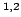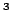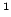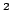Statistica Sinica 18(2008), 617-631

MINIMUM DISTANCE INFERENCE IN UNILATERAL

AUTOREGRESSIVE LATTICE PROCESSES

Marc G. Gentonand Hira L. KoulUniversity of Geneva,Texas A &M University andMichigan State University

Abstract: This paper discusses two classes of minimum distance estimators of the underlying parameters and their robust variants in unilateral autoregressive lattice models. The paper also contains an asymptotically distribution free test for symmetry of the error distribution and a goodness-of-fit test for fitting an error distribution. A lack-of-fit test for the hypothesis that the given process is doubly geometric based on the least absolute deviation residuals is also briefly analyzed. A simulation study that investigates some small sample properties of the proposed estimators and their robustness is included. It shows that some of the proposed estimators are more efficient than the least squares estimator at non-normal error distributions. We also study the empirical level and power of the test of a doubly geometric process at various error distributions. The proposed methodology is then applied to a data set of yields from an agricultural experiment.

Key words and phrases: Doubly geometric process, efficiency, non-Gaussian spatial model, Pickard process, quadrant autoregressive process, robustness, weighted empirical processes.